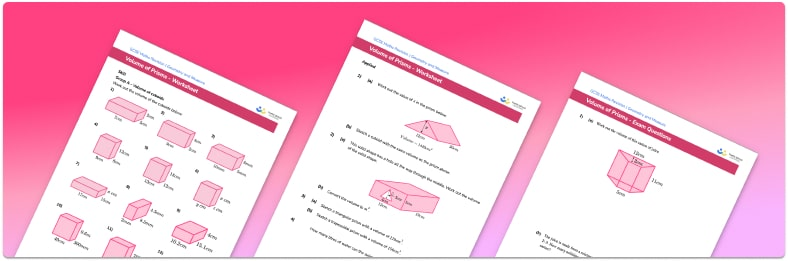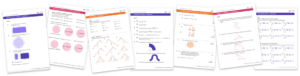# Volume Of Prisms Worksheet• Section 1 of the volume of prisms worksheet contains 27 skills-based volume of a prism questions, in 3 groups to support differentiation
• Section 2 contains 4 applied volume of a prism questions with a mix of word problems and deeper problem solving questions
• Section 3 contains 3 foundation and higher level GCSE exam style volume of a prism questions
• Answers and a mark scheme for all volume of a prism questions are provided
• Questions follow variation theory with plenty of opportunities for students to work independently at their own level
• All questions created by fully qualified expert secondary maths teachers
• Suitable for GCSE maths revision for AQA, OCR and Edexcel exam boards

• This field is for validation purposes and should be left unchanged.

You can unsubscribe at any time (each email we send will contain an easy way to unsubscribe). To find out more about how we use your data, see our privacy policy.

### Volume of prisms at a glance

Volume of 3D shapes is a measure of how much space there is inside the 3D shape. A prism is a 3D shape with two identical ends joined by a number of rectangular faces. The cross-section of a prism could be any polygon.

To calculate the volume of prisms we multiply the area of the cross-section by the length of the prism, text{Volume} = text{Cross-sectional Area} times text{Height} . For example, to find the volume of a trapezoidal prism, we would calculate the area of the trapezium and multiply this by the height of the prism.

Note that cuboids are a special type of prism and finding the volume of the cuboid could be done by finding the volume of a rectangular prism.

The volume of a prism is measured in units cubed, for example cm^3 or mm^3.

Looking forward, students can then progress to additional volume worksheets and other geometry worksheets, for example a simplifying expressions worksheet or simultaneous equations worksheet.For more teaching and learning support on Geometry our GCSE maths lessons provide step by step support for all GCSE maths concepts.

## Do you have KS4 students who need more focused attention to succeed at GCSE?There will be students in your class who require individual attention to help them succeed in their maths GCSEs. In a class of 30, it’s not always easy to provide.

Help your students feel confident with exam-style questions and the strategies they’ll need to answer them correctly with our dedicated GCSE maths revision programme.

Lessons are selected to provide support where each student needs it most, and specially-trained GCSE maths tutors adapt the pitch and pace of each lesson. This ensures a personalised revision programme that raises grades and boosts confidence.

Find out more